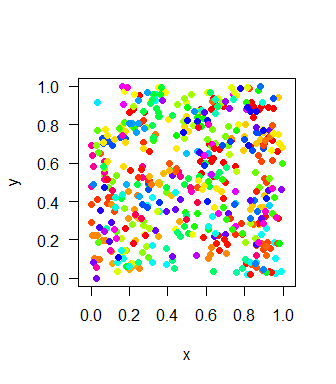1 Biodiversity components

Biodiversity in any sampling area depends on three components:

1. The total number of individuals (total abundance)
2. The relative abundances of species
3. The spatial distribution of species

mobsim provides functions to simulate communities and thereby control for all these components. This vignette first shows how to simulate non-spatial species abundance distributions (SADs) and second, how to simulate spatially- explicit community data with mobsim.

2 Simulating species abundance distributions

For this purpose mobsim provides the function sim_sad, which is a wrapper around the function rsad from the package sads. In contrast to rsad, sim_sad allows simulating communities with user-defined number of species and user-defined total number of individuals.

Here is an example for the simulation of an SAD using a log-normal model.

library(mobsim)

sad_coef = list("meanlog" = 5, "sdlog" = 0.5))
## sample_vec
## species1 species2 species3 species4 species5 species6
##       34       30       26       19       21       24
summary(abund1)
## Species abundance distribution
##
## No. of individuals:  1000
## No. of species:  100
##
## Min. abundance:  1
## Mean abundance:  10
## Max. abundance:  34

sim_sad first simulates a relative species abundance distribution according to the chosen SAD model and then samples the requested number of individuals according to this relative abundance distribution. Because of the use of a relative abundance distribution as intermediate step, in the log-normal model the mean abundance is defined by the simulated number of individuals (n_sim) divided by the number of species (s_pool). Therefore, for the log-normal model sim_sad offers also a simpler parameterization that just specifies the coefficient of variation (cv_abund) of the log-normal SAD.

summary(abund2)
## Species abundance distribution
##
## No. of individuals:  1000
## No. of species:  85
##
## Min. abundance:  1
## Mean abundance:  11.76471
## Max. abundance:  74

Obviously, the simulated community includes less species than the user-defined value of s_pool = 100. This is a consequence of the stochastic sampling from the relative abundance distribution. When some species have very low relative abundances, they might not be sampled into the simulated community. However, sim_sad offers the option fix_s_sim = TRUE, which results in the user-defined value of species in the simulation. This is implemented by adding very rare species to the community, while removing individuals from the common species. Please note that the constraint fix_s_sim = TRUE might result in deviations from the underlying theoretical SAD model.

sad_coef = list("cv_abund" = 2), fix_s_sim = T)
summary(abund2a)
## Species abundance distribution
##
## No. of individuals:  1000
## No. of species:  100
##
## Min. abundance:  1
## Mean abundance:  10
## Max. abundance:  96

The function sim_sad inherits all SAD models provided by sads::rsad. For a complete list see ?sim_sad. Here, we show an example of how to simulate a log-series SAD. It has to be noted that for some SAD models the species richness is not a direct parameter, but emerges from the other parameters. This is also true for the log-series model. Therefore, the parameter s_pool is set to NULL.

sad_coef = list("N" = 1e5, "alpha" = 20))
## sample_vec
## species1 species2 species3 species4 species5 species6
##     1837      796      813      650      488      424

Of course the simulated number of species can be easily evaluated

length(abund3)
##  123
summary(abund3)
## Species abundance distribution
##
## No. of individuals:  10000
## No. of species:  123
##
## Min. abundance:  1
## Mean abundance:  81.30081
## Max. abundance:  1837

3 Simulate spatial distributions

With mobsim random and aggregated species distributions can be simulated. This can be done in two ways. Either, simulated coordinates of individuals can be added to an observed or simulated species abundance distributions, or species abundances and distributions can be simulated simultaneously with just one function call.

3.1 Random distributions

In spatial statistics for point patterns a random distribution of points in a given area is called Poisson process. Accordingly, the function to add random coordinates to an existing species abundance distribution is called sim_poisson_coords. Here is an example of its application.

abund1 <- c(20,10,10,5,5)
comm1 <- sim_poisson_coords(abund_vec = abund1, xrange = c(0,1), yrange = c(0,1))

The community object includes x and y coordinates, as well as the species identity for every individual in the community. mobsim offers functions for exploring and plotting the community objects.

class(comm1)
##  "community"
summary(comm1)
## No. of individuals:  50
## No. of species:  5
## x-extent:  0 1
## y-extent:  0 1
##
##        x                  y                species
##  Min.   :0.002832   Min.   :0.008909   species1:20
##  1st Qu.:0.265653   1st Qu.:0.271601   species2:10
##  Median :0.533233   Median :0.420774   species3:10
##  Mean   :0.523178   Mean   :0.502736   species4: 5
##  3rd Qu.:0.776824   3rd Qu.:0.828708   species5: 5
##  Max.   :0.986101   Max.   :0.975282
plot(comm1)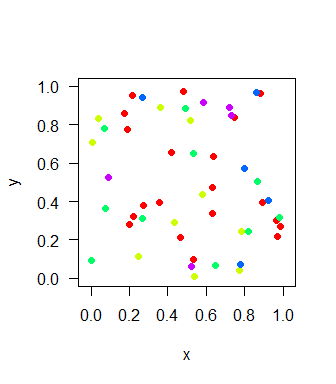As mentioned above, abundances and (random) spatial distributions can be also simulated at the same time using sim_poisson_community, which essentially calls sim_sad and sim_poisson_coords consecutively.

comm2 <- sim_poisson_community(s_pool = 20, n_sim = 200,
plot(comm2)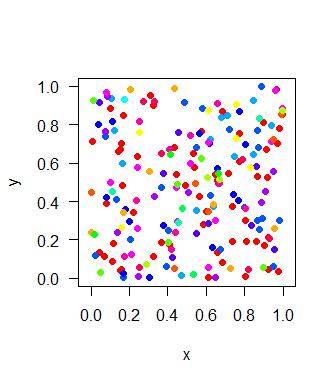3.2 Aggregated positions

Aggregated, or clustered species distributions are simulated based on the Thomas process, also known as Poisson cluster process, in mobsim (Morlon et al. 2008, Wiegand & Moloney 2014). For each species, the Thomas process first distributes a given number of mother points in the landscape. Then, offspring points are distributed around the mother points according to a bivariate Gaussian distance kernel, where the average displacement between mother and offspring points is called sigma. The offspring points constitute the final distribution of the species.

By variations in the size of clusters (sigma), the number of clusters (mother_points), and the mean number of individuals per cluster (cluster_points) the Thomas process can generate a large range of different species distributions with intraspecific aggregation.

It is important to note the each species distribution is simulated independently from the other species. That means the Thomas process in mobsim cannot be used to simulate spatial dependence between different species, i.e. interspecific aggregation or segregation.

Here is one example for a community with intraspecific aggregation:

comm3 <- sim_thomas_coords(abund_vec = abund1, sigma = 0.02)
plot(comm3)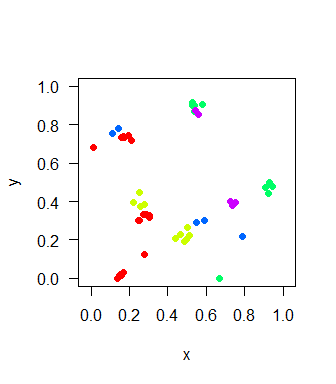First, we change the size of the clusters using the argument sigma.

comm3a <- sim_thomas_coords(abund_vec = abund1, sigma = 0.05)
par(mfrow = c(1,2))
plot(comm3)
plot(comm3a)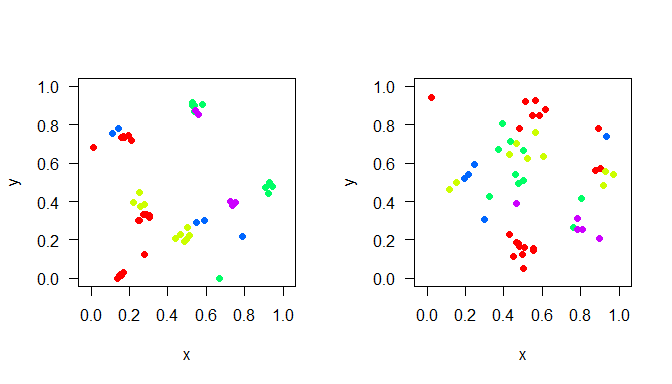Second, we change the number of clusters per species using the argument mother_points.

comm3b <- sim_thomas_coords(abund_vec = abund1, sigma = 0.02, mother_points = 1)
par(mfrow = c(1,2))
plot(comm3)
plot(comm3b)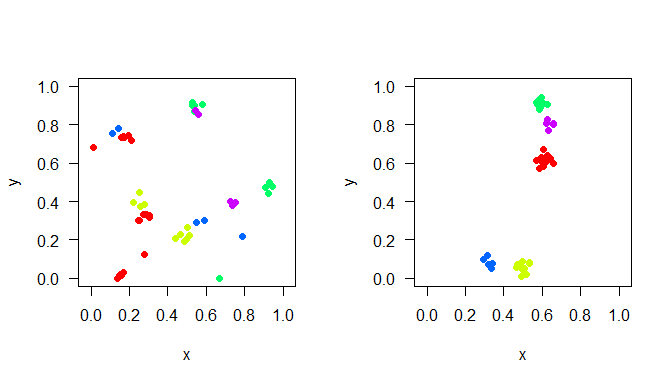Third, we change the average number of points (i.e. individuals) per cluster using the argument cluster_points.

comm3c <- sim_thomas_coords(abund_vec = abund1, sigma = 0.02, cluster_points = 5)
par(mfrow = c(1,2))
plot(comm3)
plot(comm3c)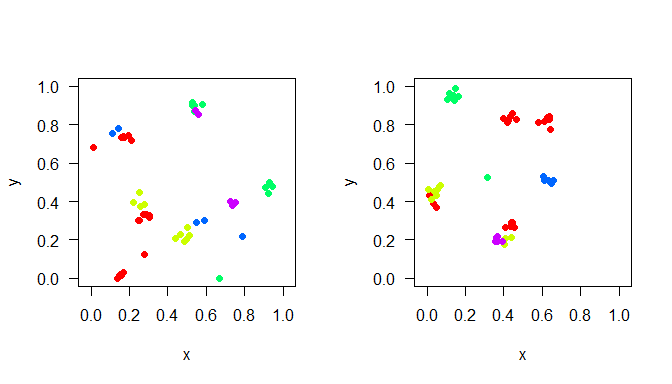Each of these parameters can be either set to the same equal value for all species as in the examples before, or individually for all species by providing a vector with a length equal to the number of species. For example, each species can has its specific number of clusters.

comm4 <- sim_thomas_coords(abund_vec = abund1, sigma = 0.02,
mother_points = c(5,4,3,2,1))
plot(comm4)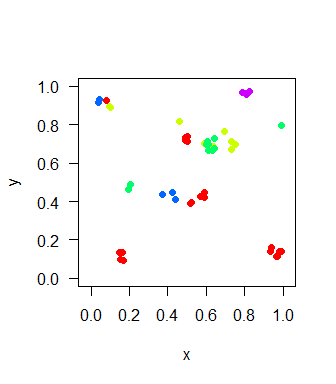Please note that there can be clusters with zero individuals, so the simulated number of clusters does not necessarily match the parameter settings.

In analogy to random distributions, there is a function to simulate abundances and aggregated distributions at the same time.

comm5 <- sim_thomas_community(s_pool = 100, n_sim = 500, sad_type = "lnorm",
sad_coef = list(cv_abund = 1),sigma = 0.05)
plot(comm5)